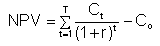# Answer to Question #6530 in Economics of Enterprise for LaMarcus Streeter

Question #6530
2. Leak Inc. forecasts the free cash flows (in millions) shown below. If the weighted average cost of capital is 11% and FCF is expected to grow at a rate of 5% after Year 2, what is the Year 0 value of operations, in millions? Assume that the ROIC is expected to remain constant in Year 2 and beyond (and do not make any half-year adjustments). Year: 1 2 Free cash flow: -\$50 \$100 a. \$1,456 b. \$1,529 c. \$1,606 d. \$1,686 e. \$1,770
1
2012-02-16T09:32:12-0500

Using the dividend growth model we have: C2 = FCF of year 3 / (WACC - Growth). FCF of year 3 = 100*(1+0.05) = 105. Thus, C2 = 105 / (11%-5%) = 1,750.
Now we need to calculate NPV:where t - the time of the cash flow; r - the discount rate (the rate of return that could be earned on an investment in the financial markets with similar risk.); the
opportunity cost of capital; Ct is the net cash flow (the amount of cash, inflow minus outflow) at time t.

NPV = (50)/(1+0,11) + 100/(1+0,11)^2 + 1750/(1+0,11)^2 = 1 456, 46.

Need a fast expert's response?

Submit order

and get a quick answer at the best price

for any assignment or question with DETAILED EXPLANATIONS!